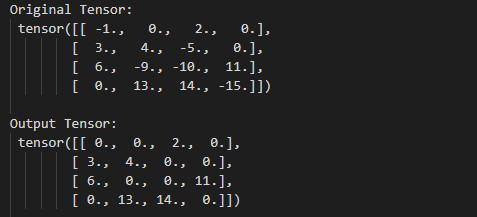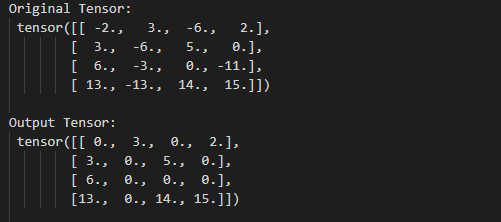Open in App
Not now

# How to Apply Rectified Linear Unit Function Element-Wise in PyTorch?

• Last Updated : 02 Jun, 2022

In this article, we are going to see How to Apply Rectified Linear Unit Function Element-Wise in PyTorch in Python. We can Rectify Linear Unit Function Element-Wise by using torch.nn.ReLU() method.

## torch.nn.ReLU() method

In PyTorch, torch.nn.ReLu() method replaces all the negative values with 0 and all the non-negative left unchanged. The values of the tensor must be real only. we can also do this operation in-place by using inplace=True as a Parameter. before moving further let’s see the syntax of the given method.

Syntax: torch.nn.ReLU(inplace=False)

Parameters:

• inplace: This parameter is use when we want to do this operation in-place. Default value of inplace is False.

Example 1:

The following program is to understand how to compute the Rectified Linear Unit Function Element-Wise.

## Python

 `# Import the required library ` `import` `torch ` `import` `torch.nn as nn ` ` `  `# define a tensor ` `input` `=` `torch.tensor([[``-``1.``, ``0.``, ``2.``, ``0.``], ` `                      ``[``3.``, ``4.``, ``-``5.``, ``0.``], ` `                      ``[``6.``, ``-``9.``, ``-``10.``, ``11.``], ` `                      ``[``0.``, ``13.``, ``14.``, ``-``15.``]]) ` ` `  `print``(``" Original Tensor: "``, ``input``) ` ` `  `# Apply Rectified Linear Unit Function  ` `# Element-Wise ` `Rel ``=` `torch.nn.ReLU() ` `Output ``=` `Rel(``input``) ` ` `  `# display result ` `print``(``" Output Tensor: "``, Output) `

Output:Example 2:

The following program is to understand how to Apply Rectified Linear Unit Function with inplace=True.

## Python

 `# Import the required library ` `import` `torch ` `import` `torch.nn as nn ` ` `  `# define a tensor ` `input` `=` `torch.tensor([[``-``2.``, ``3.``, ``-``6.``, ``2.``], ` `                      ``[``3.``, ``-``6.``, ``5.``, ``0.``], ` `                      ``[``6.``, ``-``3.``, ``0.``, ``-``11.``], ` `                      ``[``13.``, ``-``13.``, ``14.``, ``15.``]]) ` ` `  `print``(``" Original Tensor: "``, ``input``) ` ` `  `# Apply Rectified Linear Unit Function  ` `# Element-Wise Do this operation  ` `# in-place ` `Rel ``=` `torch.nn.ReLU(inplace``=``True``) ` `Output ``=` `Rel(``input``) ` ` `  `# display result ` `print``(``" Output Tensor: "``, Output) `

Output:My Personal Notes arrow_drop_up
Related Articles